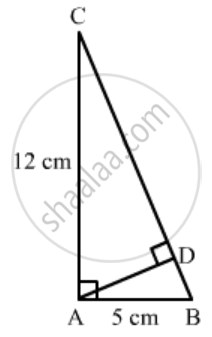Advertisement Remove all ads

# In a ∆Abc, ∠A = 90°, Ab = 5 Cm and Ac = 12 Cm. If Ad ⊥ Bc, Then Ad = 13 2 C M 60 13 C M 13 60 C M 2 √ 15 13 C M - Mathematics

MCQ

In a ∆ABC, ∠A = 90°, AB = 5 cm and AC = 12 cm. If AD ⊥ BC, then AD =

#### Options

• $\frac{13}{2}cm$

• $\frac{60}{13}cm$
• $\frac{13}{60}cm$
• $\frac{2\sqrt{15}}{13}cm$
Advertisement Remove all ads

#### Solution

Given: In ΔABC ∠ A=90^o, AD⊥ BC,, AC = 12cm, and AB = 5cm.

To find: AD

We know that the ratio of areas of two similar triangles is equal to the ratio of squares of their corresponding sides.In ∆ACB and ∆ADC,

$\angle C = \angle C$         (Common)

$\angle A = \angle ADC = 90^\circ$
∴ ∆ACB ~ ∆ADC     (AA Similarity)
(AD)/(AB)=(AC)/(BC)
AD=(ABxxAC)/BC
AD=(12xx5)/(13)
AD=60/13
We got the result as b
Concept: Triangles Examples and Solutions
Is there an error in this question or solution?
Advertisement Remove all ads

#### APPEARS IN

RD Sharma Class 10 Maths
Chapter 7 Triangles
Q 13 | Page 132
Advertisement Remove all ads

#### Video TutorialsVIEW ALL 

Advertisement Remove all ads
Share
Notifications

View all notifications

Forgot password?
Course